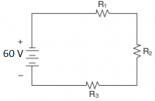The circuit in the given figure is a series circuit. We know that the same current flows through all the resistors connected in series. The equivalent resistance of the given circuit = R1+R2+R3 = 10+10+20 = 40 ohms. $$I = 60 \div 40 = 1.5$$